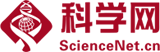##帐号 自动登录 找回密码 密码 注册
 搜索 热搜: 科学 论文
Richard Bellman,Introduction to Matrix Analysis 注释及题解

$$\dim (U_1+U_2)=\dim U_1+\dim U_2-\dim (U_1\cap U_2)$$ 证明: 采用数学归纳法.当$\dim U_2=0$时，易得$U_2=\{0\}$.此时维数定理显然成立. 设$\dim U_2=n$时，维数定理成立.则当$\dim U_2=n+1$时，令$(v_1,\cdots,v_n,v_{n+1})$为$U_2$的一组基.若$v_{n+1}\in U_1$,则dim (U_1+U_2)=dim (U_1+Span(v ...

A geometrical proof of the rearrangement inequality

In this post,we give a geometrical proof of the rearrangement inequality via dot product of two vectors. For vectors $\mathbf{OA}=(a_1,\cdots,a_n)$ and $\mathbf{OB}=(b_1,\cdots,b_n)$ in $\mathbf{R}^n$,the dot product of $\mathbf{OA}$ and $\mathbf{OB}$ is denoted by $\mathbf{OA}\cdot\mathbf{OB}$,w ...

GMT+8, 2021-10-18 10:34